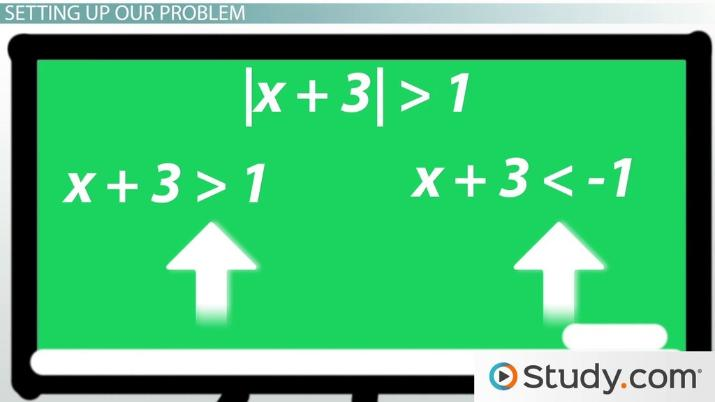# The Absolute-Value Inequality: Definition & Example

Lesson Transcript
Instructor: Yuanxin (Amy) Yang Alcocer

Amy has a master's degree in secondary education and has been teaching math for over 9 years. Amy has worked with students at all levels from those with special needs to those that are gifted.

Absolute value inequalities are types of problems that may seem complicated from the outset but are relatively simple to solve with the right understanding. Take a closer look at the definitions of the absolute value and the absolute value inequality, followed by an example of how to set up and solve these types of problems. Updated: 09/30/2021

## The Absolute Value

Before we talk about what an absolute value inequality is, let's talk about what an absolute value is. When we take the absolute value of something, we are trying to find the distance that value is from 0. For example, the absolute value of 5 is 5 since it is 5 away from 0. How about the absolute value of -5? What do you think that is? It is 5 as well because -5 is also 5 away from 0. Since we're talking math here, we also have a symbol for the absolute value and that is a vertical line on either side of the value as in |5| and |-5|.

We can solve these absolute values easily since we know that both are 5 away from 0. So, we know that |5| = 5 and |-5| = 5. If our problem was |x| = 5, then we already know that our answer is 5 and -5. Here's a trick question: Can we have |x| = -5? What do you think? Is it possible that the absolute value of something could equal a negative number? No, it's not, because we don't have a negative distance. So, if you ever see an absolute value equaling a negative number, you can right away say that the problem is false and can't be solved since the absolute value of anything can never be negative.

Now let's talk about absolute value inequalities.An error occurred trying to load this video.

Try refreshing the page, or contact customer support.

Coming up next: The Quadratic Formula: Definition & Example

### You're on a roll. Keep up the good work!

Replay
Your next lesson will play in 10 seconds
• 0:02 The Absolute Value
• 1:31 The Absolute Value Inequality
• 2:28 Setting Up Our Problem
• 3:27 Solving Our Problem
• 4:51 Lesson Summary
Save Save

Want to watch this again later?

Timeline
Autoplay
Autoplay
Speed Speed

## The Absolute Value Inequality

An absolute value inequality is a problem with absolute values as well as inequality signs. We can have problems like |x + 3| > 1. We have four different inequality signs to choose from. We have less than, greater than, less than or equal to and greater than or equal to. So, our absolute value inequalities can have any one of these four signs.

The process to solve them is the same regardless of the sign. But you do have to pay attention to what type of sign it is, as you will be taking that into account when you solve. Just like our regular absolute values, we can't have our absolute value being a negative number. So, a problem such as |x + 3| < 0 or less than a negative number will not be possible and cannot be solved.

## Setting Up Our Problem

Now let's see about solving an absolute value inequality. We will work with the inequality |x + 3| > 1. To set up our problem for solving, we need to write two problems from our one absolute value inequality. We do this by writing one problem exactly as we see the absolute value inequality but without the absolute value signs. The next one we will write will be almost the same except we are flipping the inequality sign around and changing the right side to the negative version.

So, for our absolute value inequality, our two problems are x + 3 > 1 and x + 3 < -1. Notice how I've flipped the inequality around in the second problem, and I've changed my right side to the negative version. If the right side is already negative, then we write the positive since the negative of a negative is a positive. Once we've done this, we are ready to proceed and find our answers.

To unlock this lesson you must be a Study.com Member.

### Register to view this lesson

Are you a student or a teacher?